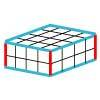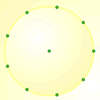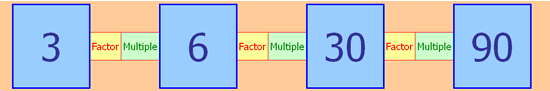You may also likeRound and Round the Circle

What happens if you join every second point on this circle? How about every third point? Try with different steps and see if you can predict what will happen.Making Cuboids

Let's say you can only use two different lengths - 2 units and 4 units. Using just these 2 lengths as the edges how many different cuboids can you make?How many DIFFERENT quadrilaterals can be made by joining the dots on the 8-point circle?

Factor-multiple Chains

Age 7 to 11 Challenge Level:

Here is an example of a factor-multiple chain of four numbers:Can you see how it works? Perhaps you could make some statements about some of the numbers in the chain using the words "factor" and "multiple".

In these chains, each blue number can range from $2$ up to $100$ and must be a whole number.

You may like to experiment with this spreadsheet which allows you to enter numbers in each box. Perhaps you can make some more chains for yourself.

What are the smallest blue numbers that will make a complete chain?
What are the largest blue numbers that will make a complete chain?
What numbers cannot appear in any chain?
What is the biggest difference possible between two adjacent blue numbers?
What is the largest and the smallest possible range of a complete chain? (The range is the difference between the largest and smallest values.)